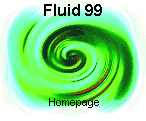ScheduleComputational Fluid Dynamics

________Centro Internacional de Matemática
Observatório Astronómico da Univ. Coimbra
July 12-17, Coimbra (Portugal)

Coordinator: A. Quarteroni

1. Domain Decomposition Methods in Fluid Dynamics
A. Quarteroni (Poli. Milano, Italy and EPFL, Lausanne, Switzerland).

2. Multilevel Methods in Fluid Dynamics
C. Canuto
(Poli. Torino, Italy);

3. Spectral Methods for Incompressible and Compressible Flows
Y. Maday (ASCI-CNRS, Orsay and Univ. Paris VI, France);

4. An Introduction to Numerical Methods for Fluid Dynamics and Upwind Schemes
B. Perthame (Ecole Normale Supérieure, Paris, France);Domain Decomposition Methods in Fluid Dynamics

A. Quarteroni
(Politecnico di Milano, Italy
and EPFL, Lausanne, Switzerland)

For the numerical simulation of partial differential equations , the computational efficiency of a certain numerical method can be enhanced by resorting to different strategies. Among these are the geometrical partition of the computational domain into subdomains, and the use of the so-called multifield approach.

The former allows the reduction of the original problem to a family of subproblems of reduced size. At this stage, we will show the extent at which domain decomposition algorithms can provide valuable computational tools within parallel computing environments.

The latter can be regarded as a way for simplifying both the mathematical description of the problem at hand and the associated numerical algorithm, via the combination of multiple kinds of differential models and numerical schemes. The resulting approach has a "heterogeneous" nature that can allow a substantial reduction of the computational complexity without affecting the accuracy of the computed solution.

The scope of these lectures is to describe domain decomposition principles, and some interesting instances of multifield methods in fluid dynamics. We will consider advection-diffusion processes for either compressible and incompressible flow problems, and show how domain decomposition strategies can be conveniently adopted to simplify our problem.

Lecture 1. Principles of domain decomposition methods for elliptic problems
Lecture 2. Domain decomposition methods for time-dependent problems
Lecture 4. Application to compressible flows
Lecture 5. Application to incompressible flowsMultilevel Methods in Fluid Dynamics

C. Canuto (Politecnico di Torino, Italy)

Multilevel methods provide an efficient tool to approximate functions by summing-up details of decreasing importance. Thus, they are naturally suited to tackle complex problems in fluid dynamics, whenever highly structured flows are encountered.

In the numerical discretization of boundary value problems, multilevel methods allow for an easy preconditioning and/or compression of the related matrices, as well as for designing optimal adaptative strategies; the initial limitation of these methods to simple geometries has been removed by the active research of the last few years.

Multilevel ideas have been employed in handling the classical difficulties arising in the numerical simulation of incompressible flows, namely, pressure instabilities in the Stokes operator and convective instabilities at high Reynolds numbers. In addiction, turbulence analysis and turbulence modeling based on wavelet expansions offer new perspectives in the simulation of turbulent flows.

Lectures:

• Definition and basic properties of multilevel methods. Examples: hierarchical finite elements, wavelets.
• Complex geometries and domain decomposition methods.
• The Stokes problem: satisfying or circumventing the inf-sup condition.
• The Navier-Stokes equation: flow at high Reynolds number and turbulence modeling.Spectral Methods for Incompressible and Compressible Flows

(ASCI-CNRS, Orsay
and Univ. Paris VI, France)

Among the numerical methods to tackle the approximation of partial differential equations and more specifically in fluid dynamics, variational methods have these days quite a success. They are indeed rather versatile and allow to couple with domain decomposition techniques and parallel algorithm rather easily.

Spectral methods enter in this familly of approximations and allow to offer a high order approximation of the solution. This means that, when the solution is worth the full use of the high order, spectral methods achieve a given accuracy with a smaller amount of degrees of freedom in turn providing a lower cost of the resolution. These methods allow also to derive a better accuracy than other lower order methods.

These methods, based on the proper use of polynomial approximations, rely now on firm theoretical background and recently much attention has been paid to the derivation of fast and efficient solvers.

The purpose of these lectures is to provide the tools for the approximation elliptic partial differential equation then of the Navier Stokes equations and also to give some basics on the way to generalize them for the solution of nonlinear conservations laws where the solution is known to be most often discontinuous.

The last lectures will allow to understand how to couple these approximations with domain decomposition techniques in order both to handle complex geometries and to take benefit of parallel opportunities.

Lectures

1. Basics of spectral methods
2. Spectral methods for the Navier-Stokes equations
3. Spectral methods for conservation laws
4. Spectral element methods and implementation aspects
5. The mortar element method in case of spectral approximationsAn Introduction to Numerical Methods for Fluid Dynamics and Upwind Schemes

B. Perthame
(Ecole Normale Supérieure, Paris, France)

This course will present an introduction to the numerical methods used in fluid dynamics equations and more generally in hyperbolic systems. It intends to present the basis of the finite volume method (conservative schemes, Riemann solvers, entropy properties, convergence rates), but also to raise several more recent developments: fluctuation, splitting method in two space dimensions, central schemes, relaxation methods... we will also raise the question of 'equilibrium schemes' which aim to solve precisely hiperbolic systems with source terms (river flows for instance).

Contents

1. Conservation laws, gas dynamics systems, entropies.
2. Finite volume methods (the 1D scalar case)
3. Finite volumes for gas dynamics
4. Fluctuation splitting schemes
5. Central schemes
6. Relaxation schemes
7. Source terms and equilibrium schemes